How to Learn in 24 Hours?The Rapid Learning Movie

 Need Help? M-F: 9am-5pm(PST): Toll-Free: (877) RAPID-10 US Direct: (714) 692-2900 Int'l: 001-714-692-2900 24/7 Online Technical Support: The Rapid Support Center Secure Online Order:Need Proof? Testimonials by Our Users

 Rapid Learning Courses: MCAT in 24 Hours (2015-16) USMLE in 24 Hours (Boards) Chemistry in 24 Hours Biology in 24 Hours Physics in 24 Hours Mathematics in 24 Hours Psychology in 24 Hours SAT in 24 Hours ACT in 24 Hours AP in 24 Hours CLEP in 24 Hours DAT in 24 Hours (Dental) OAT in 24 Hours (Optometry) PCAT in 24 Hours (Pharmacy) Nursing Entrance Exams Certification in 24 Hours eBook - Survival Kits Audiobooks (MP3)

 Tell-A-Friend: Have friends taking science and math courses too? Tell them about our rapid learning system.Home »  Mathematics »  High School Algebra 2

Rational Functions

 Topic Review on "Title": Rational functions:  Any function which can be written of the form: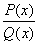where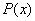and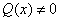are polynomials. Domain of a rational function: The set of points where the function is defined. Vertical asymptote: A line of the form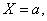such that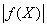takes arbitrarily large values near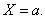Horizontal asymptote: A line of the form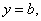such that if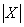tends to infinity,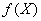tends to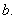The sum of rational functions: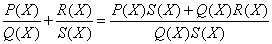. The product of rational functions: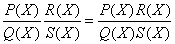. Simple functions Simple functions are rational functions that cannot be simplified any further. The division of rational functions: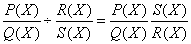.

Rapid Study Kit for "Title":
 Flash Movie Flash Game Flash Card Core Concept Tutorial Problem Solving Drill Review Cheat Sheet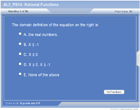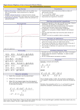"Title" Tutorial Summary : This tutorial describes rational functions and their properties. The domain of rational functions is described with the use of horizontal and vertical asymptotes. The degrees of the two functions composing of a rational function are important to find the asymptotes of rational functions. This tutorial describes the operation of rational functions with the use of examples. A rational function can be simplified by factoring the numerator function of rational functions and the denominator function of rational functions. Solving rational equations involves comparing the degrees and evaluating both functions so simplification can be achieved as much as possible.

 Tutorial Features: Specific Tutorial Features: • Rational functions are reduced using simplification techniques. • The asymptotes of rational functions are shown in some of the examples. Series Features: • Concept map showing inter-connections of new concepts in this tutorial and those previously introduced. • Definition slides introduce terms as they are needed. • Visual representation of concepts • Animated examples—worked out step by step • A concise summary is given at the conclusion of the tutorial.

 "Title" Topic List: Rational functions and their basic definitions The domain of rational functions Rational functions and their asymptotes FormulasThe operations of rational functions Simple rational functions Simplification of rational functionsPolynomial equations Polynomial equations and their solutions

See all 24 lessons in High School Algebra 2, including concept tutorials, problem drills and cheat sheets:
Teach Yourself High School Algebra 2 Visually in 24 Hours